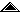Universidad de Costa Rica Escuela de Ciencias de la Computación e Informática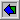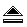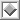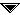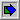# `mnyfmt.c`: A simple function to format money or currency amounts

## AbstractThe simple `mnyfmt(char*,char,long long,unsigned)` C function can be used to format money and currency amounts using picture clauses that mimic those of the Cobol language. As this implementation is self-contained, it can be used to avoid both C and C++ locales because it provides a viable alternative to format numbers in many practical situations.

## IntroductionIt is easy to describe what formatting money is: it is the task of obtaining a string that corresponds to the value of an amount that is stored in a numeric (binary) variable. For example, if the amount is `-2455.87003` one possible formatting is `"\$**-2,455.88"` and another is `"(€ 2.455,87)"`; note that it is a valid strategy to truncate or to round up a floating point value. The complexity of this task increases because there are many choices for rounding [WIKI-2012a], as well as different currencies and symbols to represent them: (`'\$'`, `'¥'`, `'€'`, `'£'`, `'₡'`, etc.). Moreover, countries use a different number of decimal fractions for their money: most European currencies have 2 decimals cents, but many Arab currencies require 3 decimals for the fractional parts.

At first sight it appears that inserting commas `','` every `3` digits and later swapping them with dots `'.'` when needed does the job, but there are cases that can appear weird to many: in India digits in money quantities are grouped in pairs, but the second group can contain up to `3` digits [WIKI-2012b]:

For example, the amount 3,25,84,729.25 is read as three crore(s), twenty-five lakh(s), eighty-four thousand, seven hundred and twenty-nine rupees and twenty-five paise.

To me, this means that every application must define many details when dealing with money values. There are just way too many rounding choices and way too many formatting alternatives, none of which can be tagged as the more general or more appropriate. In the C and C++ world the solution has been to use locales, where currency information is stored. In an explanation on how to deal with them, P.J. Plauger writes:

"Committee X3J11 put all this stuff in the C Standard because IBM said that's what the world needed. Shawn Elliott, the IBM representative to the C standards committee, showed us a report that was truly mind-boggling. It showed all the ways that people write monetary and non-monetary values around the world. I didn't know whether to be more impressed by the variety that the IBM researchers unearthed or by the effort they made to unearth it." [Plauger-1991].

In his paper, Plauger also provided a function named `_Fmtval()` to use locales for formatting currency. However, in a later article he said that his function was "... messy and hard to read ..." [Plauger-1998]. This was really discouraging to me, as Plauger is a C/C++ expert with notorious knowledge on the subject of locales. I asked myself; "Is it possible to format money quantities without resorting to locales?" (Probably, I should not say this, but my drive to find a different solution comes from my dislike for the complexities of locales in both C and C++).

When I learned programming, I was first taught Fortran (for "scientific applications") and later Cobol. I had to use a lot of picture clauses [WIKI-2012c] in my programs: these are simple to define, use, and provide many formatting options. Each format digit `'9'` in picture clause `"999,999.99"` gets substituted by the corresponding number. Also, the monetary sign can "float" toward the more significant digit: this means that the picture clause `"\$\$\$,\$\$9.99"` will result in `"\$22,555.00"` for a big number, or in `"\$22.00"` for a smaller one, as the leading `'\$'` signs become white space as needed. These patterns have worked well for decades, but they are not part of the C++ or C library. Why is that? I do not know the answer, but nonetheless I decided to write a function that mimics Cobol's approach to formatting.

## Simpler is betterI named my function `mnyfmt()`, which is a 6 letter acronym, inspired on the name of the `strlen()` function that first mentions the name of the object it acts on (a C string implemented as a zero terminated character array), and uses the last 3 letters to describe the action it does (calculate the length of the string). I thought of calling the function `fmtmny()`, but I decided that mentioning that name in this article would be enough for search engines to hit on `mnyfmt()` -- neither name is "taken" which is a good thing.

My second challenge was to figure out how to round numbers [WIKI-2012a]. I discovered that truncation is just one of more than a dozen options and I also discovered that bankers use a special rounding scheme called "round half to even", where rounding goes to the closer integer number, but halves always round to an even number. For example, `-12.63` rounds to integer `-13` and `-12.50` rounds to `-12` but `+13.50` rounds to `14`. Handling so many rounding schemes is overwhelming, which lead me to decide to leave the problem aside. Hence, my function does not receive a floating point value, but instead uses 2 integer parameters: one is the integer part of the number to format, and the other is the number of digits in the fractional part. Programmers must round their values before invoking `mnyfmt()`: the invocation reads `mnyfmt("99.99",'.',1250,2)` to yield `"12.50"` (with 2 decimal digits).

My first implementation was a C++ template function receiving a `std::string<>` argument. However, I discovered that I was not using any template nor `std::string<>` functionality: this lead me to replace the C++ `std::string<>` with a regular zero terminated character array. I also added a parameter to mark the fractional separator. In most of my test programs I used only commas `','` and dots `'.'`, but there are situations where other fractional separators are useful. At that point the prototype for the function was this:

``` char* mnyfmt(char *fmtstr, char dec, long intpart, int CE); ```
 ```{{ // test.overwrite typedef struct struct_overwrite { long align; // probably aligned char bytes_8; // 64 bits: probably aligned int int_neg; // -1 usually has all its bits equal to 1 } overwrite; overwrite o = { 0, {'1','2','3','4','5','6','7','\0' }, -1 }; assertTrue( 8-1==strlen(o.bytes_8) && o.int_neg == -1 ); strcpy( o.bytes_8, "1234567.." ); // 2 more bytes... assertTrue( 9==strlen(o.bytes_8) ); assertFalse( o.int_neg == -1 && "Adjacent memory overwritten " ); assertTrue( o.int_neg != -1 ); assertTrue( CHAR_BIT == 8 && "8 bits bytes required" ); }} ```
Figure 1

I decided to use only one (`char*`) argument because I know that C strings are problematic when dealing with limited memory. For example, in Figure 1 field `bytes_8[]` can only hold 8 characters: when 10 are copied into it, the last 2 will overwrite whatever values are stored after `bytes_8[]`, in this case changing the value of the field `int_neg`. The value stored within `bytes_8[]` will not seem wrong, but the operation will change other value (or values): this is what makes this type of error particularly difficult to catch, as it causes failures that behave in strange ways that oftentimes are not consistent. When the string that contains the picture clause is also the place where the formatted value will reside, the programmer needs to check that this variable is large enough. However, for most applications a string of 96 or 128 bytes will be big enough because numbers with more than 40 digits are rare; 14 trillion requires (only) 16 digits, including 2 digits for the fraction, which makes a 48 or a 96 string at least twice as big as it is needed to hold the formatted value.

It is a well known programming best practice to build any program along with its test data [Beck-2002]. The assertions `assertTrue()` and `assertFalse()` used in most examples in this article mimic those of JUnit (the test framework for Java [BG-1998]) and are further explained in [DiM-2008]: in here they just output the condition tested when it fails. These 2 macros are defined in header file `uUnit.h`. Besides clarifying the code, these examples come directly from the test program in file `mnyfmtts.c`. For example, whenever the assertion `assertTrue(1==2)` is executed in line 63 of the `mnyfmtts.c` file, it puts the following message on the standard output:

```=\_fail: 1==2
=/ (63) mnyfmtts.c
```
 ```{{ // test.sgn.ptr char *sgn, fmtstr[mnyfmt_size]; // Picture clause strcpy( fmtstr , "999.999.999,99" ); if (( sgn = mnyfmt( fmtstr , ',', 12345678988,2 ) )) { assertTrue( eqstr( fmtstr , "123.456.789,88" ) ); assertTrue( eqstr( fmtstr , sgn ) && (fmtstr == sgn) ); } strcpy( fmtstr , "999.999.999,99" ); if (( sgn = mnyfmt( fmtstr , ',' , -10245587,2 ) )) { assertTrue( eqstr( fmtstr , "00-.102.455,87" ) ); assertTrue( eqstr( sgn , "-.102.455,87" ) ); if ( (*sgn=='-') && ('.'==*(sgn+1)) ) { ++sgn; *sgn='-'; } assertTrue( eqstr( sgn , "-102.455,87" ) ); } { assertFalse( 0077 == 77 ); // 0077 is an octal literal assertTrue( 0077 == 8*7+7 ); // 0077 == 63 decimal } }} ```
Figure 2

Earlier versions of `mnyfmt()` returned a pointer to the formatted string, (mimicking `strcpy()`'s behavior), but it turns out that it is more useful if a pointer to the first significant digit is returned, as it is shown in Figure 2, so that the same picture clause `"999.999.999,99"` can be used to format a small value like `1235.87` or a huge one like `123456789.88` (which reaches hundreds of millions). Also, `mnyfmt()` always uses character `'-'` to represent the negative sign, and it gets placed on top of the corresponding format character `'9'`: the only character in the format string that ever gets changed is format character `'9'`. As expected, `eqstr()` compares 2 C strings and returns `true` only if they are equal. Octal number literals always begin with digit 0; this can lead to errors if a number literal is used, as it is shown in the lower part of Figure 2, where the program source code is `"0077"` but the compiler interprets (correctly) the base 8 literal number as `63`.

As `mnyfmt()` simply substitutes each format character `'9'` with the corresponding digit, sometimes the result begins with a decimal separator, as it happened in the example shown in Figure 2 where the returned result `"-,102.455,87"` contains a comma after the `'-'` sign. This special case should be handled by the programmer as there is no generalized solution that can be applied by `mnyfmt()`.

 ```{{ // test.example // (2^128 < 10^40) && (2*40<96) ==> char holds a char *sgn, fmtstr,mem; // 128 bit integer unsigned CE, ten_pow_CE; CE = 2; ten_pow_CE = 100; // 10^CE -> "Currency Exponent" strcpy( mem, "USD\$ " ); // Picture clause strcpy( fmtstr , "99,999,999.99999" ); if (( sgn = mnyfmt( fmtstr , '.' ,-10245587,CE ) )) { assertTrue( eqstr( fmtstr , "0-,102,455.87000") ); if ( (*sgn=='-') && (','==*(sgn+1)) ) { ++sgn; *sgn='-'; } assertTrue( eqstr( sgn, "-102,455.87000") ); strcat( mem , sgn ); assertTrue( eqstr( mem, "USD\$ -102,455.87000") ); } else { assertFalse( "ERROR [???]: " "-102,455.87000" ); } }} ``` ```{{ // test.PITFALL char *sgn, fmtstr[mnyfmt_size], buffer[mnyfmt_size]; strcpy( buffer, "USD\$ " ); // Picture clause strcpy( fmtstr , "99,999,999" ); if (( sgn = mnyfmt( fmtstr , '.' ,-102455,87 ) )) { assertFalse( "ERROR [CE should be 0 not " ",87" "]" ); } else { assertTrue( sgn == 0 ); // NULL means mnyfmt() returns ERROR  } strcpy( fmtstr , "99,999,999.9999" ); if (( sgn = mnyfmt( fmtstr , '.' ,-1024550077,4 ) )) { assertTrue( eqstr( sgn , "-,102,455.0077" ) ); // handle the "-," problem if ( (*sgn=='-') && (','==*(sgn+1)) ) { ++sgn; *sgn = '-'; // put '-' sign in a nice place } assertTrue( eqstr( sgn , "-102,455.0077" ) ); } }} ```
Figure 3

The implementation of `mnyfmt()` is quite straightforward: it locates the decimal fraction character, and backs up substituting the format character `'9'` with the corresponding digit, then does the same forward with the decimal fraction (dot `'.'` in Figure 3). Any other characters remain untouched, and only the format characters that come immediately after the decimal fraction character are substituted. To avoid using floating point values, only integer numbers are use to represent numeric values, where their decimal part digits are the less significant digits in the integer number. For example, the value `-102455.87` would be converted to integer number `-10245587` where the last 2 digits are decimals. In this case, the scale factor is `10^2==100` and the value to format is `-102455.87*(10^2)`. When `mnyfmt()` fails for any reason, it returns `(char*)(0)`: as it is shown in Figure 3, every invocation to `mnyfmt()` requires checking that a non null value is returned.

The number of decimals "CE" cannot be arbitrary. It must be a number in the range `[0..7]`: in Figure 3 (marked `test.PITFALL`) value `87` for the Currency Exponent "CE" forces `mnyfmt()` to return error `NULL==(char*)(0)`. Note also that even though `-10245587`, the integer value to format in `test.example`, should be formated using only 2 decimals because the last parameter "CE" `mnyfmt()` is 2, as the fractional part in the format string has 5 decimal digit holds `"99,999,999.99999"`, the 2 decimals 87 get formatted as `".87000"`, filling the non significant decimal digits with 0.

 ```{{ // test.too.small char *sgn, fmtstr[mnyfmt_size]; strcpy( fmtstr , "999.99999" ); if (( sgn = mnyfmt( fmtstr , '.' , 24558700,4 ) )) { // never executed ==> buffer too small // 2455 has 4>3 digits [999.] } assertTrue( sgn == 0 ); assertTrue( eqstr( fmtstr , "999.99999") ); }} ``` ```{{ // test.few.decimals char *sgn, fmtstr[mnyfmt_size]; strcpy( fmtstr , "999,999,999.9999" ); if (( sgn = mnyfmt( fmtstr , '.' ,-124550077,2 ) )) { assertTrue( eqstr( sgn , "-1,245,500.7700" ) ); assertFalse( eqstr( sgn , "-102,455.00" ) ); assertFalse( eqstr( sgn , "-102,455.0077" ) ); } }} ```
Figure 4

In Figure 4, within `test.too.small`, the value returned by `mnyfmt()` is the null pointer `(char*)(0)` because the integer part of the value to format `2455` requires space for 4 digits, but the picture clause has only 3 format characters `'9'` before the decimal separator `'.'` (as expected, passing a null pointer to `mnyfmt()` also results in a null pointer return). The value stored in `fmtstr` remains unchanged. Within `test.few.decimals`, even though the format string `"999,999,999.9999"` has 4 decimal positions, as parameter "CE" is 2, `mnyfmt()` interprets number `1024550077` as `10245500.77` (with only 2 decimals and separator `'.'`). This is a bit misleading. The trailing and non significant digits ones get filled with `'0'`. If the number should be interpreted as a 4 decimal number, then the last parameter "CE" should be 4.

 ```{{ // test.parentheses char *sgn, fmtstr[mnyfmt_size], buffer[mnyfmt_size]; strcpy( buffer, "USD\$ " ); strcpy( fmtstr , "9,999,999.999" ); if (( sgn = mnyfmt( fmtstr , '.' ,-102455087,3 ) )) { if ( *sgn=='-' ) { // put parentheses around the formatted value if (','==*(sgn+1)) { ++sgn; *sgn='-'; } // skip comma strcat( buffer , "(" ); strcat( buffer , sgn ); strcat( buffer , ")" ); assertTrue( eqstr( buffer, "USD\$ (-102,455.087)") ); } else { strcat( buffer , sgn ); } } }} ```
Figure 5

More interesting formatting can be achieved with `mnyfmt()`. For example, in Figure 5 a second variable called `buffer` is used to put parentheses around the formatted value if it is negative.

 ```{{ // test.asterisks char *sgn, fmtstr[mnyfmt_size]; strcpy( fmtstr , "\$9,999,999.999" ); if (( sgn = mnyfmt( fmtstr , '.' , -455870,3 ) )) { if ( (*sgn=='-') && (','==*(sgn+1)) ) { ++sgn; *sgn='-'; } assertTrue( eqstr( sgn , "-455.870") ); for ( --sgn; (sgn!=fmtstr ); --sgn ) { *sgn = '*'; // avoid writing over "\$" } assertTrue( eqstr( fmtstr , "\$*****-455.870" ) ); } }} ```
Figure 6

Sometimes the money amount must fill the whole picture clause and its leading non significant digits must be displayed as an asterisk `'*'`. Figure 6 is an example of this.

 ```{{ // test.modf char *sgn, fmtstr[mnyfmt_size]; double intdouble, fractdouble; long intpart, fracpart; unsigned CE, ten_pow_CE; CE = 5; ten_pow_CE = 100*1000; // 10^CE fractdouble = modf( 2455.87 , &intdouble ); intpart = intdouble; // 2455 fracpart = (unsigned)(fractdouble*ten_pow_CE); // .86000 { assertFalse( fracpart == 87000 && "???"); assertTrue( fracpart == 86999 && "!!!"); // binary rounding... } strcpy( fmtstr , "[[ 999,999.99999 ]]" ); if (( sgn = mnyfmt( fmtstr , '.' , intpart*ten_pow_CE+fracpart,CE ) )) { assertTrue( eqstr( fmtstr , "[[ 002,455.86999 ]]" ) ); assertTrue( eqstr( sgn , "2,455.86999 ]]") ); { // std::round_toward_infinity fracpart = (unsigned)(ceil(fractdouble*100))*1000; strcpy( fmtstr , "[[ 999,999.99999 ]]" ); assertTrue( fracpart == 87000 && "!!!"); if (( sgn = mnyfmt( fmtstr , '.' , intpart*ten_pow_CE+fracpart,5 ) )) { assertTrue( eqstr( sgn, "2,455.87000 ]]") ); } } } }} ``` ```{{ // test.SCALE #define SCALE(f,CE) ( (mnyfmt_long) ( (f) * 1.0e##CE ) ) // beware of truncation  char *sgn, fmtstr[mnyfmt_size]; double val_double = -102455.87; strcpy( fmtstr , "99,999,999.99999" ); if (( sgn = mnyfmt( fmtstr , '.' ,SCALE(val_double,2),2 ) )) { assertTrue( eqstr( fmtstr , "0-,102,455.86000") ); if ( (*sgn=='-') && (','==*(sgn+1)) ) { ++sgn; *sgn='-'; } assertTrue( eqstr( sgn, "-102,455.86000") ); } // rounding and trucations yields .86 instead of .87 else { assertFalse( "ERROR [???]: " "-102,455.86000" ); } }} ```
Figure 7

Dealing with floating point values requires some care. In the example in Figure 7 the `(double)` value to format is taken apart into its integer and fractional parts using standard function `modf()`. The floating point value written in the program is `2455.87`, but the fractional part calculated by `modf()` is `86`, not `87`, as the binary representation of this number is not exact in machines that use IEEE 754 binary floating point arithmetic [WIKI-2012d]. To get the expected result a rounding strategy must be applied: in this case it is rounding up. The non format characters `"[[ ]]"` that surround the picture clause are left untouched by `mnyfmt()`. A macro can be used to convert floating point currency values into integers to be formatted, as it is shown in the lower part of Figure 7 where macro the `SCALE(f,CE)` is defined. However, the integer conversion from floating point can truncate the value in extrange ways, because in this example the souce code reads `-102455.87` but macro `SCALE()` produces the wrong scaled integer `-10245586`.

 ```{{ // test.limit char *sgn, fmtstr; #ifndef MNYFMT_NO_LONG_LONG mnyfmt_long max = LONG_LONG_MAX; strcpy( fmtstr , "999,999,999,999,999,999,999" ); // 9,223,372,036,854,775,807 if ( 9223372036854775807LL == LONG_LONG_MAX ) { if (( sgn = mnyfmt( fmtstr , ' ' , max,0 ) )) { assertTrue( eqstr( "9,223,372,036,854,775,807", sgn ) ); } } else { assertFalse( "BEWARE: (long long) is not 8 bytes wide" ); } #endif { mnyfmt_long max = LONG_MAX; strcpy( fmtstr , "999,999,999,999" ); // 2,147,483,647 if ( 2147483647L == LONG_MAX ) { if (( sgn = mnyfmt( fmtstr , ' ' , max,0 ) )) { assertTrue( eqstr( "2,147,483,647", sgn ) ); } } else { assertFalse( "BEWARE: (long) is not 4 bytes wide" ); } } }} ```
Figure 8

The final implementation of `mnyfmt()` can be compiled by any version of a C compiler. This makes the function even more useful because it is available to a wider audience. Even older C++ compilers support the `(long long)` data type, which gets implemented at least as a 64 bit binary number that has enough range to represent most money quantities. To make it possible to use `mnyfmt()` in compilers that do not have this type, macro `MNYFMT_NO_LONG_LONG` is used to define `mnyfmt_long` (the type of the integer part of the number), as `(long)` instead of `(long long)` as shown in Figure 8.

 ```{{ // test.fract.zero char *sgn, fmtstr[mnyfmt_size]; // The fraction 643/2136 approximates log10(2) to 7 significant digits. int N = ( ( CHAR_BIT * sizeof(int) - 1 ) * 643 / 2136 ) * (10000*10000); strcpy( fmtstr , "999,999,999,999.999999" ); if (( sgn = mnyfmt( fmtstr , '.', -N,0 ) )) { if ( (*sgn=='-') && (','==*(sgn+1)) ) { ++sgn; *sgn='-'; } assertTrue( eqstr( fmtstr ,"00--900,000,000.000000" ) ); assertTrue( eqstr( sgn , "-900,000,000.000000" ) ); assertFalse( eqstr( fmtstr , "00--455.000000012" ) ); assertFalse( eqstr( sgn , "-455.000000012" ) ); } }} ```
Figure 9

The number of trailing zeroes in the fractional part does change the formatted value, as it is shown in Figure 9. The signature for the final version of `mnyfmt()` is:

```#ifndef MNYFMT_NO_LONG_LONG
typedef long long mnyfmt_long;
#else
typedef long mnyfmt_long;
#endif

char* mnyfmt(char *fmtstr, char dec, mnyfmt_long intpart, unsigned CE);
```

## Further detailsThe test program `mnyfmtts.c` contains all the program segments shown in this article and a few more. It can be used to understand the behavior of `mnyfmt()` in many situations beyond those described here.

Many C library functions come in several flavors, one for each type. For example, functions `lround()`,`lroundf()`, `lroundl()` `llround()`,`llroundf()` and `llroundl()` are versions for the same rounding function whose name changes because they return a different type of integer and take a different type of floating point. For `mnyfmt()` it is not necessary to have these many versions because conversion to the wider integer type is always provided by the compiler. It is also unnecessary to provide versions that use the wider characters types, as (`wchar_t`), because picture clauses use single byte characters (`char`) that can be converted easily into those other types.

 ```{{ // Convert a char[] into a wchar_t[] char *src, chBuff; wchar_t *dst, *wcBuff; strcpy (chBuff, "Convert me to (wchar_t)"); wcBuff = (wchar_t*)( malloc( sizeof(chBuff)*sizeof(wchar_t) ) ); for ( dst=wcBuff,src=chBuff; (*dst=*src); ++dst,++src ) {} // ... C++ will let you use more sophisticated stuff free(wcBuff); }} ```
Figure 10

The relationship between types `(char)` and `(wchar_t)` is difficult to manage; for example, the C language standard does not specify the exact type for `(wchar_t)` which can be 2 or 4 bytes wide (or even a single byte). This passage, taken from [WIKI-2012e], describes some of the complexities:

"ANSI/ISO C leaves the semantics of the wide character set to the specific implementation but requires that the characters from the portable C execution set correspond to their wide character equivalents by zero extension."

Moreover, type `(char)` can be either `(signed char)` or `(unsigned char)`. As most format picture clauses for `mnyfmt()` use only characters form the 103 portable character set, which the POSIX standard requires to be present in any character set, the easier path to follow is to use the `(char)` type for picture clauses and a direct conversion into `(wchar_t)` wherever it is needed, as shown in Figure 10.

 ```{{ // test.minus.max long long_min = -LONG_MAX-1; assertTrue( long_min<0 ); long_min = -long_min; assertTrue( long_min<0 && "?????" ); assertTrue( long_min == -long_min ); }} ```
Figure 11

Most processors use 2's complement binary arithmetic that behaves strangely in the smallest negative value, as it is shown in Figure 11. This is why `mnyfmt()` fails to calculate the correct formatted result for this case (and returns a null pointer).

 ```{{ // test.stop char *sgn, fmtstr[mnyfmt_size]; strcpy( fmtstr , "999,999.9999919,one9." ); if (( sgn = mnyfmt( fmtstr , '.', 245587,2 ) )) { if ( (*sgn=='-') && (','==*(sgn+1)) ) { ++sgn; *sgn='-'; } assertTrue( eqstr( fmtstr , "002,455.8700019,one9." ) ); assertTrue( eqstr( sgn , "2,455.8700019,one9.") ); } }} ```
Figure 12

The behavior of `mnyfmt()` is different when formatting the integer instead of the fractional part of the number because all leading non significant format characters in the integer part get replaced by character `'0'`, but only those that immediately follow the decimal separator get changed. This is why in Figure 12 the character `'1'` in the format string stops the substitution leaving the remaining format characters unchanged. This code also shows a bad programming practice as there is no check to ensure that variable `sgn` is not null. This is an error that can cause a program failure when the null pointer returned by `mnyfmt()` gets used to change a value in memory. Enclosing every invocation to `mnyfmt()` in and `if(){}` statement is necessary to avoid this pitfall.

 ```{{ // test.rupee char *sgn, fmtstr[mnyfmt_size]; char *p; strcpy( fmtstr , "99,99,99,99,99,99,99,999.99" ); // LONG_LONG_MAX == 92,23,37,20,36,85,47,758.07 // 3,25,84,729.25 // 19 digits: 12 34 56 78 90 12 34 567 89 if (( sgn = mnyfmt( fmtstr , '.' , 3258472925LL,2 ) )) { assertTrue( eqstr( sgn , "3,25,84,729.25" )); } strcpy( fmtstr,"99,99,99,99,99 crores 99 lakhs 99,999 rupees.99 paise" ); if (( sgn = mnyfmt( fmtstr , '.' , 3258472925LL,2 ) )) { for ( p=sgn; *p!='.'&&*p!=0; ++p ) {} // advance p to dec '.' *p = ' '; // blank the dot: "rupees." ==> "rupees." } assertTrue( eqstr( sgn , "3 crores 25 lakhs 84,729 rupees 25 paise" )); // Rp3,25,84,729.25 is read as three crore(s), twenty-five lakh(s), // eighty-four thousand, seven hundred and twenty-nine rupees and // twenty-five paise. // - http://en.wikipedia.org/wiki/Indian_rupee#Numeral_system }} ```
Figure 13

The example in Figure 13 shows that a picture clause can be used to format rupee [()] amounts where words are also included. This code looks for the decimal separator `'.'` and replaces it with a blank space `' '` to achieve a more convincing result.

 ```{{ // test.times char *sgn, fmtstr[mnyfmt_size]; strcpy( fmtstr , "99/99/9999" ); if (( sgn = mnyfmt( fmtstr , 000, 9272002,0 ) )) { assertTrue( eqstr( fmtstr , "09/27/2002" ) ); assertTrue( eqstr( sgn , "9/27/2002" ) ); } strcpy( fmtstr , "99:99:99" ); if (( sgn = mnyfmt( fmtstr , '?', 21435,0 ))) { assertTrue( eqstr( fmtstr , "02:14:35") ); assertTrue( eqstr( sgn , "2:14:35") ); } }} ```
Figure 14

Picture clauses can be used to format almost any type of numeric values. In Figure 14 shows how to handle dates and hours, but many more applications are possible. First, the decimal separator used is (octal number) zero. In the second example, `mnyfmt()` uses the end of string character `'\0'` as decimal separator because the question mark `'?'` does not appear in the format string.

 ```{{ // test.no.strcpy char *sgn, fmtstr[mnyfmt_size]; strcpy( fmtstr , "9,999." ); if (( sgn = mnyfmt( fmtstr , '.' , 2455,0 ) )) { assertTrue( eqstr( sgn , "2,455." ) ); } if (( sgn = mnyfmt( fmtstr , '.' , 1400,0 ) )) { // never executed: missing strcpy() // no char in "2,455." is a format char } else { assertFalse( eqstr( fmtstr , "1,400." ) ); assertTrue( eqstr( fmtstr , "2,455." ) ); // ??? assertTrue( "BEWARE: missing strcpy()" ); // ??? { strcpy( fmtstr , "9,999." ); sgn = mnyfmt( fmtstr , '.' , 1400,0 ); assertTrue( eqstr( sgn , "1,400." ) ); } } }} ```
Figure 15

Figure 15 illustrates a possible mistake any programmer could incur on: failure to copy the format string into the formatting variable before invoking `mnyfmt()`. Many programmers will choose to place their currency formatting logic inside functions that are tailored to each application in order to prevent mistakes like this one, which are simple to fix, but could be difficult to spot in a big program.

The double parentheses in the `if(){}` statement that contains the invocation to `mnyfmt()` might seem odd, but it is a good programming practice suggested by the compiler: "warning: suggest parentheses around assignment used as truth value".

```/* good */   if  ( sgn = mnyfmt( fmtstr , '.', 2455,2 ) )  { // ...
/* BETTER */ if (( sgn = mnyfmt( fmtstr , '.', 2455,2 ) )) { // ...
```

It is easier for me to use the `'9'` digit as the format character for `mnyfmt()`, because it functions as digit `'9'` in a Cobol picture clause. However, someone might want to change it to `'#'` or to `'@'`. This requires recompilation to change the value for macro `mnyfmt_format_char` defined in header file `mnyfmt.h` (the test cases should also be changed accordingly). There is no danger of losing the source code for this implementation, as it is on the public domain under the Boost.org license:

`http://www.boost.org/LICENSE_1_0.txt`

These programs compile correctly using either the C or the C++ compiler. To compile the C source using the C++ compiler, override the file's extension (the GNU gcc compiler does this with option `-x`: `gcc -x c++`).

## ConclusionsFunction `mnyfmt()` does the job because good enough currency formatting can be done with it. Further internationalization can be dealt with using a library like ISOMON that provides simple access to ISO currency data [Castedo-2012]. A C++ programmer can choose to pack `mnyfmt()`inside a more sophisticated `std::string<>` function because picture clauses require a little bit of care:

• Make sure that the format character array is big enough to hold the format string:
`if ( sizeof(fmtstr) <= 1+strlen("999.999.999") ) { // error ...`
• Remember to copy the format string into the format array:
`strcpy(fmtstr,"999.999.999");`
• Always check the returned value to avoid dereferencing a null pointer:
`if (( sgn = mnyfmt( fmtstr , '.' , 1400,2 ) )) { // ...`
• Remember that negative numbers sometimes have the `'-'` sign before a comma or a dot.
`if ( (*sgn=='-') && (','==*(sgn+1)) ) { ++sgn; *sgn='-'; }`
`   "-,102,455.87"``"-102,455.87"`
`if ( (*sgn=='-') && ('.'==*(sgn+1)) ) { ++sgn; *sgn='-'; }`
• Never use negative numbers for the fractional part:
`if (( sgn = mnyfmt( fmtstr , '.' , 1400,-1 ) )) { // BOOMMM!!!`
• Be careful when rounding. Maybe it is a good idea to take a look into [Goldberg-1991].
```/// Round to integer, rounding halfway cases away from zero
double round(double val) { // unbiased rounding
//   return ( (val>0.0) ? floor(val+0.5) :  ceil(  val-0.5) );
return ( (val>0.0) ? floor(val+0.5) : -floor(-val+0.5) );
}
```
 ``` char* mnyfmt(char *fmtstr, char dec, mnyfmt_long intpart, unsigned fractpart); ``` Formats and stores in `fmtstr` the money amount. Before invocation , the formatting pattern (picture clause) is stored in result string `fmts tr`. To avoid using `(double)` values that have many round off problems, the parameter for this function is an integer scaled to `10^C E` digits. For example, when using `CE== 2` digits, the monetary value `"\$2, 455.87"` is representa d by the integer `'245 587'`, and if `CE== 4` digits are used, the integer value would be `'245 58700'`. The (integer) value to format is `moneyval`. Overwrites `fmtstr` with the formatted value. On error, leaves `fmtstr` untouched and returns `(char*)(0)`. If the `fmtstr` does not have enough format characters `'9'` for the integer part to format, of if the `'-'` cannot fit on top of a `'9'` character, `fmtstr` remains untouched and the value returned is `(char*)(0)`. The valid range for CE, the 'currenct exponent', is [0..6] [ a CE of 7 or bigger leaves `fmtstr` untouched and the value returned is `(char*)(0)` ]. The first occurrence of the character `dec` is the decimal fraction separator (usually `'.'` or `','`). When the decimal fraction separator character `dec` does not appear in `fmtstr` it is assumed to be `'\0'` (end of string character). After the `dec` separator all the leading consecutive `'9'` format characters are substituted with the corresponding digit from the decimal part in `moneyval`, using digit zero `'0'` as fill character. All digits that inmediatly follow the decimal fraction separator are changed either to zero or to the corresponding digit taken from the decimal part in `moneyval`. Both the integer part and the fractional part are filled with the digits that correspond to its position. This means that a format string like `"9999.9999"`wild yield `"0123.8700"`as result when `moneyval`==1238700 and `CE==4`. Characters trailing after the `dec` separator that are not the `'9'` format digit are left untouched. All format characters `'9'` appearing before the decimal separator `dec` will be replaced by digit zero `'0'` if the corresponding digit in `moneyval` is not significant. When `moneyval` is negative, the `'-'` sign will be place over the `'9'` immediately before the more significant digit. Non format characters in `fmtstr` are left untouched. The negative sign always is `'-'` and it is always placed on top of the corresponding format character. Returns `(char*)(0)` when the formatted value does not fit within `strlen(fmtstr)` characters. Returns a pointer to the first significant digit in the formatted string, within `fmtstr` or to the `'-'` sign if the formatted value is negative. Before storing the format string in `fmtstr`, the programmer must ensure that `fmtstr` is big enough to hold the format string. Remarks: This routine basically substitutes each `'9'` character in `fmtstr` for its corresponding decimal digit, or `'0'` when it is not a significant digit. All other characters within `fmtstr` remain untouched. As it happens with many C functions, before invocation the programmer must be sure that `fmtstr` is big enough to copy on it the complete format string, as otherwise memory beyond `fmtstr` would be overwritten. There is no \c (wchart_t) version for this function, as it is meant to place digits in a formatting string. After placement, the result string can be converted to other forms.
Figure 16

## AknowledgmentsAlejandro Di Mare, Miguel Angel Prieto and David Chaves made valuable suggestions that helped improve earlier versions of this work. The spelling and grammar were checked using the `http://spellcheckplus.com/` tool. The Graduate Program in Computación e Informática, the Escuela de Ciencias de la Computación e Informática and the Universidad de Costa Rica provided funding for this research.

## Source code`mnyfmt.zip` source code:
`http://www.di-mare.com/adolfo/p/mnyfmt/mnyfmt.zip`
`mnyfmt()`: A simple function to format money or currency amounts
`[.h.html.c]` `[.h][.c]` `[.h.txt.c]`
`http://www.di-mare.com/adolfo/p/mnyfmt/mnyfmt_8c.html`
`mnyfmtts()`: test→`mnyfmt()`:
`[html...txt...c]`
`http://www.di-mare.com/adolfo/p/mnyfmt/mnyfmtts_8c.html`
`uUnit.h`: `assertTrue()` && `assertFalse()`
`[html...txt...h]`
`http://www.di-mare.com/adolfo/p/mnyfmt/uUnit_8h.html`

`gcov` instruction coverage:
`http://www.di-mare.com/adolfo/p/mnyfmt/gcov`

Doxygen:
`ftp://ftp.stack.nl/pub/users/dimitri/doxygen-1.7.5.1-setup.exe`

## References`           ` [Beck-2002] Beck, Kent: Test driven development, Addison Wesley, Reading, MA, USA, 2002. [BG-1998] Beck, Kent & Gamma, Erich: JUnit Test Infected: Programmers Love Writing Tests, Java Report, 3(7):37-50, 1998.       `http://junit.sourceforge.net/doc/testinfected/testing.htm` [Castedo-2012] Ellerman, Castedo: ISOMON: C/C++ library for money and ISO 4217 currency codes.       `http://isomon.castedo.com/`       `http://code.google.com/p/isomon/source/browse/` [DiM-2008] Di Mare, Adolfo: `BUnit.h`: Un módulo simple para aprender prueba unitaria de programas en C++ , X Simposio Internacional de Informática Educativa (ADH'08) realizado del 1 al 3 de octubre 2008, Salamanca, España, I.S.B.N.: 978-84-7800-312-9, pp425-430, octubre 2008.       ``` http://www.di-mare.com/adolfo/p/BUnit.htm ``` [Goldberg-1991] Goldberg, David: What Every Computer Scientist Should Know About Floating-Point Arithmetic, CACM Computing Surveys, Vol 23, No 1, March, 1991.       `http://citeseerx.ist.psu.edu/viewdoc/summary?doi=10.1.1.102.244` [Plauger-1991] P.J. Plauger: Standard C: Formatting Monetary Values, The C Users Journal, June 1991.       `http://www.drdobbs.com/article/print?articleId=184402377` [Plauger-1998] P.J. Plauger: Standard C/C++: The Facet moneypunct, The C Users Journal, March 1998.       `http://www.drdobbs.com/article/print?articleId=184403475` [WIKI-2012a] Wikipedia, the free encyclopedia: Rounding, 2012.       `http://en.wikipedia.org/wiki/Rounding` [WIKI-2012b] Wikipedia, the free encyclopedia: Indian rupee, 2012.       `http://en.wikipedia.org/wiki/Indian_rupee` [WIKI-2012c] Wikipedia, the free encyclopedia: Picture clause, 2012.       `http://en.wikipedia.org/wiki/Picture_clause` [WIKI-2012d] Wikipedia, the free encyclopedia: IEEE 754-1985, 2012.       `http://en.wikipedia.org/wiki/IEEE_754-1985` [WIKI-2012e] Wikipedia, the free encyclopedia: Wide character, 2012.       `http://en.wikipedia.org/wiki/Wide_character`

## Indice[-] Abstract  Introduction  Simpler is better  Further details  Conclusions  Aknowledgments  Source code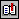BibliografíaIndice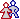Acerca del autor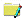Acerca de este documentoPrincipioIndiceFinal

## Acerca del autor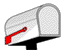Adolfo Di Mare <adolfo@di-mare.com>
## Acerca de este documentoReferencia: Di Mare, Adolfo: `mnyfmt.c`: A simple function to format money or currency amounts, Technical Report 2012-02-ADH, Escuela de Ciencias de la Computación e Informática, Universidad de Costa Rica, 2012. Internet: ``` http://www.di-mare.com/adolfo/p/mnyfmt.htm ```       Google™ Translate ``` http://www.di-mare.com/adolfo/p/mnyfmt/mnyfmt.zip``` ``` http://www.di-mare.com/adolfo/p/mnyfmt.pdf     ```       Google™ Translate [old version] ``` http://www.di-mare.com/adolfo/p/mnyfmt_old.htm ```       Google™ Translate [old version] See Also: ``` http://www.drdobbs.com/cpp/232700238 http://www.drdobbs.com/article/print?articleId=232700238 ``` Autor: Adolfo Di Mare ``` ``` Contacto: Apdo 4249-1000, San José Costa Rica Tel: (506) 2511-8000       Fax: (506) 2438-0139 Revisión: ECCI-UCR, February 2015 Visitantes: